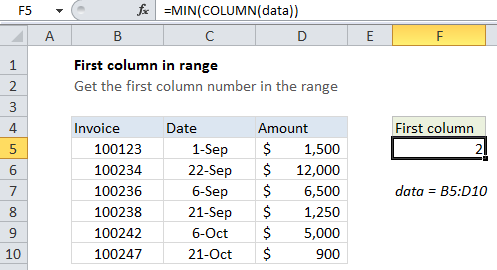## Excel Office

Excel How Tos, Tutorials, Tips & Tricks, Shortcuts

# How to get first column number in range in Excel

You can get the first column (i.e. the starting column number) in a range with a formula based on the COLUMN function.

## Formula

`=MIN(COLUMN(range))`## Explanation

In the example shown, the formula in cell F5 is:

`=MIN(COLUMN(data))`

where data is a named range for B5:D10

### How this formula works

When given a single cell reference, the COLUMN function returns the column number for that reference. However, when given a range that contains multiple columns, the COLUMN function will return an array that contains all column numbers for the range. In the example shown the array looks like this:

Worked Example:   Fibonacci Sequence in Excel

{2,3,4}

If you want only the first column number, you can use the MIN function to extract just the first column number, which will be the lowest number in the array.

Worked Example:   Calculate total hours that fall between two times in Excel

### Simple version

Entered in a single cell, the COLUMN function will display only the first column number, even when it returns an array. This means, in practice, you can often just use the COLUMN function alone:

`=COLUMN(range)`

However, inside formulas more complex formulas, it’s sometimes necessary to make sure you are dealing with only one item, and not an array. In that case, you’ll want to use MIN to pull out just the first item.

Worked Example:   Understanding Named Range, Named Constant, Name Manager in Excel Formulas